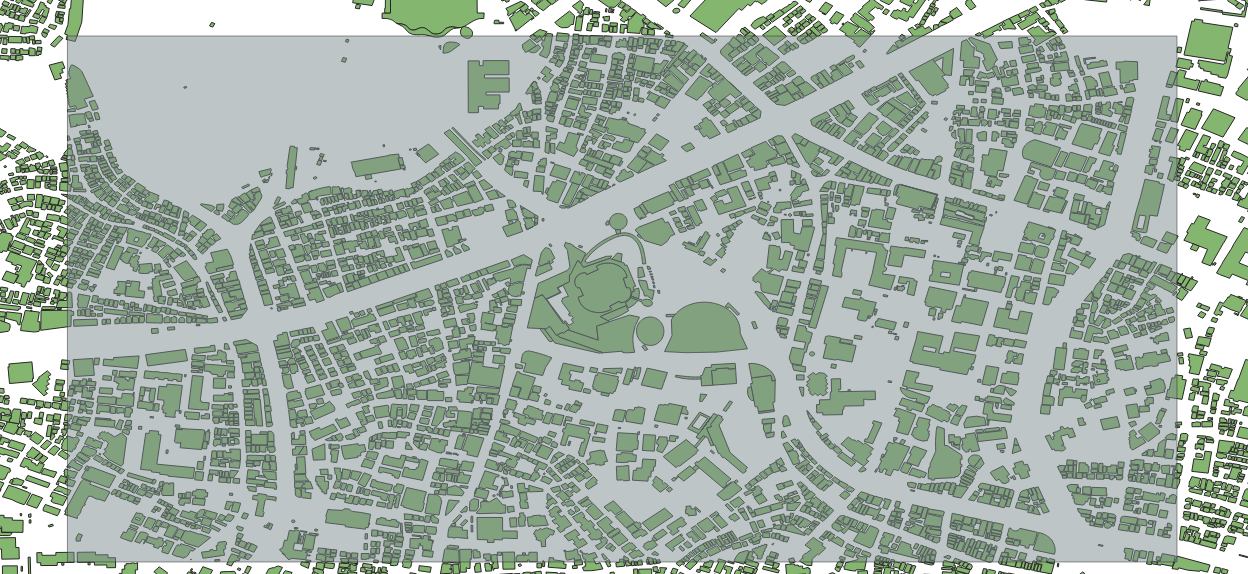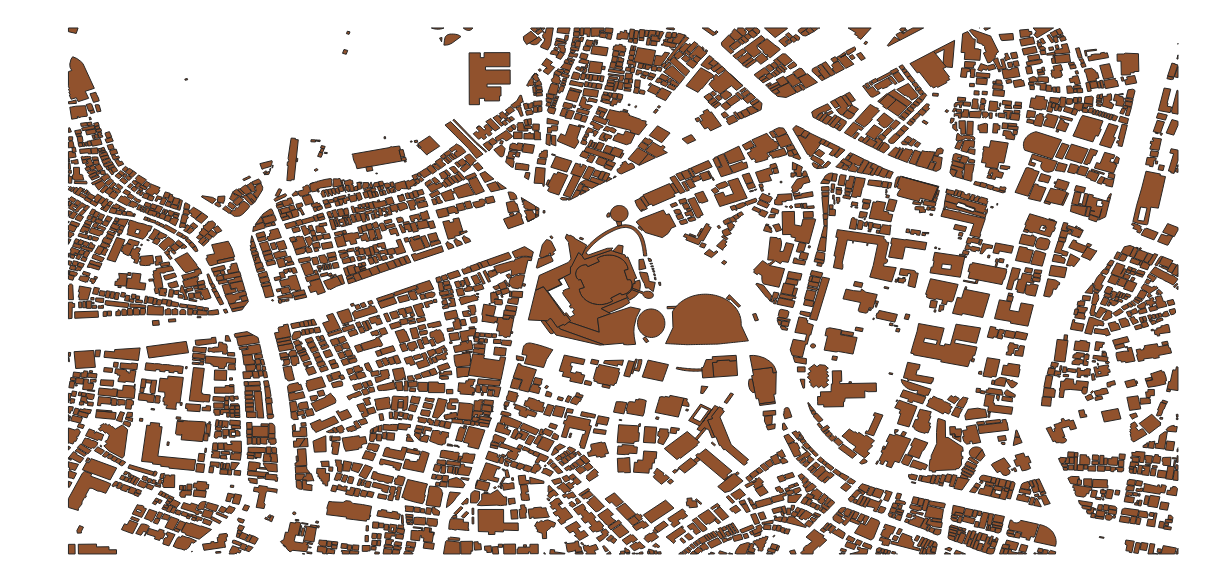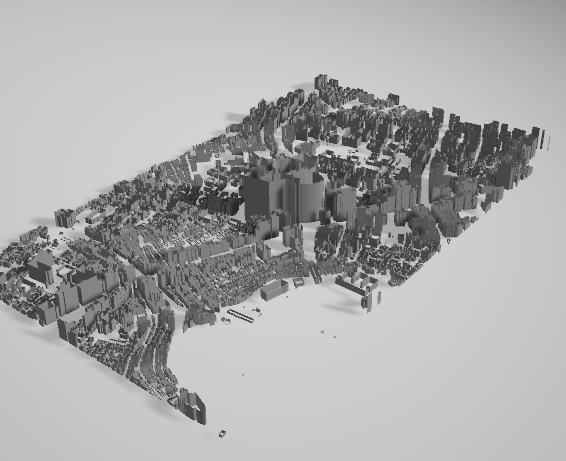# Introduction

Let's export the GIS data to an OBJ file using the Python console built into QGIS.

• For OBJ files, please present the [Wikipedia link](https://ja.wikipedia.org/wiki/Wavefront_.obj file). It's a very simple format that can be exported as text, so it's easy to handle when exporting your own.

# Cutout

First, cut out the target range. If you use the previous data as it is, it will be too huge and real-time rendering will not be able to handle it, so try cutting it out to an appropriate range. This time, I will cut out the area around Roppongi Hills. First, select "Layer"-"Create Layer"-"New Temporary Scratch Layer" from the menu, and in the dialog, select a polygon with an appropriate name and geometry type. Make sure that the new layer you added is in edit mode, and select "Edit"-"Add Rectangle"-"Add Rectangle for Area Range" from the menu. Left-click to select vertices diagonal to each other, and right-click to finish adding.In the figure, the drawing of the rectangle is made semi-transparent for easy understanding.

Next, select "Vector"-"Spatial Calculation Tool"-"Crop", select the layer of building data for input, and the layer with the rectangular area you just created for overlay, and execute it.

The area you want to export is now cropped.By the way, find the center of gravity of this rectangular area. "Vector"-"Geometry Tool"-"Center of Gravity". You can find the latitude and longitude of the center of gravity by clicking the created point with the feature information display tool.

# QGIS macro? Python console

Now, let's write this data to a 3D OBJ file using the Python console. The Python console can be called from the menu with "Plugins"-"Python Console".

# Coordinate transformation

Currently the coordinate system is working with WGS84 (probably if nothing has changed). If you start to explain the details of the coordinate system, it will be a tremendous amount, so I will omit it here. In WGS84, the coordinates are expressed in latitude and longitude. From here, convert to the XYZ 3D Cartesian coordinate system. The following method is a coordinate conversion method created by referring to the program in the book World Geodetic System and Coordinate Conversion. It is a Cartesian coordinate conversion that is as accurate as possible according to the representation of the earth in WGS84. Note that this calculation must be done with at least double precision. Python's floating point should be double, so you don't have to worry too much about it, but Unity's Vector3 class etc. have numbers in float, so be careful when porting this program. When dealing with geographic coordinates often, it's better to deal with doubles so that you don't make any extra mistakes.

``````def BLH2XYZ(b,l,h):
# WGS84
a = 6378137.0
f = 1.0 / 298.257223563

e2 = f * (2.0 - f)
N = a / math.sqrt(1.0 - e2 * math.pow(math.sin(b), 2.0))

X = (N + h) * math.cos(b) * math.cos(l);
Y = (N + h) * math.cos(b) * math.sin(l);
Z = (N * (1 - e2) + h) * math.sin(b);

return (X,Y,Z)
``````

# OBJ export

Then, create an OBJ export program by incorporating the above coordinate conversion method.

``````def BLH2XYZ(b,l,h):
# WGS84
a = 6378137.0
f = 1.0 / 298.257223563

e2 = f * (2.0 - f)
N = a / math.sqrt(1.0 - e2 * math.pow(math.sin(b), 2.0))

X = (N + h) * math.cos(b) * math.cos(l);
Y = (N + h) * math.cos(b) * math.sin(l);
Z = (N * (1 - e2) + h) * math.sin(b);

return (X,Y,Z)

layer = iface.activeLayer()
features = layer.getFeatures()

i = 0
j = 0
fp = open("test.obj",mode='w')
fp.write("g "+str(i)+"\n")

#* Change to the center of gravity of the rectangular area acquired earlier
cx = 139.72957
cy = 35.66021

ox,oy,oz = BLH2XYZ(cy,cx,0)

for feature in features:
# print(feature.id())
mp = feature.geometry().asMultiPolygon()
try:
height = int(feature['height'])*2
if height < 1:
height = 2
except:
height = 5

for polygon in mp:
for polyline in polygon:
i=i+1
prev_p_index = j

for point in polyline:
x,y,z = BLH2XYZ(point.y(),point.x(),0)
x2,y2,z2 = BLH2XYZ(point.y(),point.x(),height)
x = x - ox
y = y - oy
z = z - oz
x2 = x2 - ox
y2 = y2 - oy
z2 = z2 - oz

rx = x * math.cos(s) - y * math.sin(s)
ry = x * math.sin(s) + y * math.cos(s)

rxx = rx * math.cos(s) - z * math.sin(s)
rz  = rx * math.sin(s) + z * math.cos(s)

rx2 = x2 * math.cos(s) - y2 * math.sin(s)
ry2 = x2 * math.sin(s) + y2 * math.cos(s)

rxx2 = rx2 * math.cos(s) - z2 * math.sin(s)
rz2  = rx2 * math.sin(s) + z2 * math.cos(s)

fp.write("v "+str(ry)+" "+str(rxx)+" "+str(rz)+"\n")
fp.write("v "+str(ry2)+" "+str(rxx2)+" "+str(rz2)+"\n")
j=j+1

current = j - prev_p_index
for num in range(current):
p1 = (2*num+1)
p2 = (2*num+2)
p3 = (2*num+3)
p4 = (2*num+4)

if p3 > current*2:
p3 = p3 - current*2

if p4 > current*2:
p4 = p4 - current*2

p1 = p1 + prev_p_index*2
p2 = p2 + prev_p_index*2
p3 = p3 + prev_p_index*2
p4 = p4 + prev_p_index*2

fp.write("f "+str(p1)+" "+str(p2)+" "+str(p3)+" "+str(p1)+"\n")
fp.write("f "+str(p4)+" "+str(p3)+" "+str(p2)+" "+str(p4)+"\n")

fp.close()
print("Done")

``````

In this program, the processing is the coordinate conversion from the geographic coordinates to the orthogonal coordinates, the movement of the origin, the rotation of the whole, and the writing to OBJ.

# result